## Introduction

While teaching a class on statistical physics, I found myself unhappy with textbook derivations of the Wiener-Khinchin theorem. I worked my way to a very short derivation that is free of integral bounds manipulations and of holes, or so I believe.

In either the books by Balakrishnan (Elements of Nonequilibrium Statistical Mechanics, Ane Books, 2008), Risken (The Fokker-Planck Equation, 2nd edition, Springer-Verlag, 1989), Coffey-Kalmykov-Waldron (The Langevin Equation, 2nd edition, World Scientific, 2004) or MathWorld, I could not find a short derivation that would cleanly take into account the averaging procedure or that would not resort to splittings of the domain of integration. I present here one derivation and a numerical illustration with Python.

## Definitions¶

• We are intersted in the real-valued signal or process $\xi(t)$
• $\tilde\xi(\omega) = \int_{-\infty}^\infty dt e^{-i\omega t} \xi(t)$ is the Fourier transform of $\xi(t)$.
• Complex conjugates are denoted by a star $\tilde\xi^\ast(\omega)$
• $\xi(t) = (2\pi)^{-1} \int_{-\infty}^\infty d\omega e^{i\omega t} \tilde\xi(\omega)$ defines the inverse Fourier transform.
• The autocorrelation of $\xi(t)$ is $C(\tau) = \lim_{T\to\infty} T^{-1} \int_0^T dt \xi(t) \xi(t+\tau)$
• The spectral power density is $S(\omega) = |\tilde\xi(\omega)|^2 / (2\pi)$

## Derivation¶

We start by the definition of the autocorrelation

$$C(\tau) = \lim_{T\to\infty} T^{-1} \int_0^T dt \xi(t) \xi(t+\tau)$$

and replace $\xi(t)$ by the inverse transform of $\tilde\xi(\omega)$

$$C(\tau) = \lim_{T\to\infty} T^{-1} \int_0^T dt\int_{-\infty}^\infty \frac{d\omega}{2\pi} e^{i\omega t} \tilde\xi(\omega) \int_{-\infty}^\infty \frac{d\omega'}{2\pi} e^{i\omega' (t+\tau)} \tilde\xi(\omega')~,$$

change the order of the integrals $$C(\tau) = \lim_{T\to\infty} \int_{-\infty}^\infty \frac{d\omega}{2\pi} \int_{-\infty}^\infty \frac{d\omega'}{2\pi} T^{-1} \int_0^T dt e^{i\omega t} e^{i\omega' (t+\tau)} \tilde\xi(\omega) \tilde\xi(\omega')~,$$ $$C(\tau) = \lim_{T\to\infty} \int_{-\infty}^\infty \frac{d\omega}{2\pi} \int_{-\infty}^\infty \frac{d\omega'}{2\pi} T^{-1} \int_0^T dt e^{i(\omega+\omega') t} e^{i\omega' \tau} \tilde\xi(\omega) \tilde\xi(\omega')~.$$

The integral over $t$ will depend on the value of $\omega+\omega'$. Explicitly, the subcases are

1. $\omega+\omega'\neq 0$ Here, writing $T= n 2 \pi / (\omega+\omega') + T'$, where $0\leq T'<2 \pi / (\omega+\omega')$ we find that the integral is zero to order $\approx 1/T$.
2. $\omega+\omega'=0$ Here the integral is equal to $T$

By only keeping the non-zero contribution, we can use $\omega+\omega'=0$ and obtain $$C(\tau) = \frac{1}{2\pi} \int_{-\infty}^\infty \frac{d\omega}{2\pi} e^{-i\omega \tau} \tilde\xi(\omega) \tilde\xi(-\omega)$$ and by using the fact that for a real-valued $\xi(t)$, $\tilde\xi(-\omega)=\tilde\xi^\ast(\omega)$ and changing the variable $\omega$ to $-\omega$ in the integral $$C(\tau) = \frac{1}{2\pi} \int_{-\infty}^\infty \frac{d\omega}{2\pi} e^{i\omega \tau} \tilde\xi(\omega) \tilde\xi^\ast(\omega) = \frac{1}{2\pi} \int_{-\infty}^\infty \frac{d\omega}{2\pi} e^{i\omega \tau} |\tilde\xi(\omega)|^2$$

We have thus obtained the Wiener-Khinchin theorem that states that the autocorrelation of a signal is the inverse Fourier transform of its spectral power density divided by $2\pi$.

## Illustration¶

For illustration, I consider a periodic signal and a Ornstein–Uhlenbeck process. For the numerical evaluation of the autocorrelation using FFTs, see the dedicated blog post: http://pdebuyl.be/blog/2016/correlators.html

The "code cell" below loads the math, random, NumPy, matplotlib and SciPy libraries. There are small differences due to the fact that the signals are of finite length, though!

In :
%matplotlib inline
import math
import random
import numpy as np
import matplotlib.pyplot as plt
import scipy.signal
plt.rcParams['font.size'] = 18
plt.rcParams['figure.subplot.wspace'] = 0.25


### Periodic signal¶

Let us consider a sinusoid with pulse $\omega = 2.7$. The power spectrum will have peaks at $\pm\omega$ that would converge to Dirac deltas for an infinite time series.

The autocorrelation can be computed analytically and is a cosine. It is plotted for reference.

In :
# Define the signal

N = 1024
omega = 2.7
dt = 2*np.pi/omega/128
time = np.arange(N)*dt
xi = np.sin(omega*time)

plt.plot(time, xi)
plt.xlabel(r'$t$')
plt.ylabel(r'$\xi(t)$');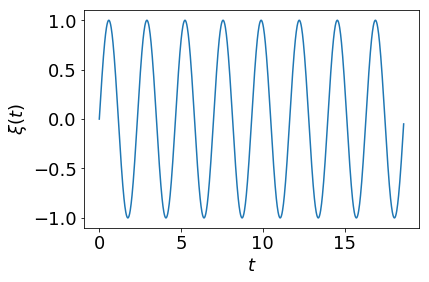In :
# Analytical value of the autocorrelation

plt.plot(time, 0.5*np.cos(omega*time))

# Compute numerically the autocorrelation via a Fourier transform

fft_cor = scipy.signal.fftconvolve(xi, xi[::-1])[N-1:]
fft_cor /= (N - np.arange(N))
plt.plot(time, fft_cor, 'k-', lw=2)

# Compute the autocorrelation via the Wiener-Khinchin theorem
# The NumPy fft routines include the 2 pi factors

psd = np.fft.fft(xi)*np.conj(np.fft.fft(xi))/N
C = np.fft.ifft(psd).real

plt.plot(time, C)

plt.xlim(0, 5*2*np.pi/omega)

plt.xlabel(r'$\tau$')
plt.ylabel(r'$C(\tau)$');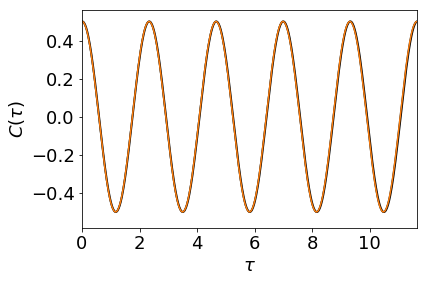In :
# Plot the spectral power density

plt.plot(np.fft.fftfreq(N, dt), psd.real)
plt.axvline(-omega/(2*np.pi), ls='--', c='k')
plt.axvline(omega/(2*np.pi), ls='--', c='k')

plt.xlim(-2, 2)
plt.xlabel(r'$\nu$')
plt.ylabel(r'$|\tilde\xi(\nu)|^2$');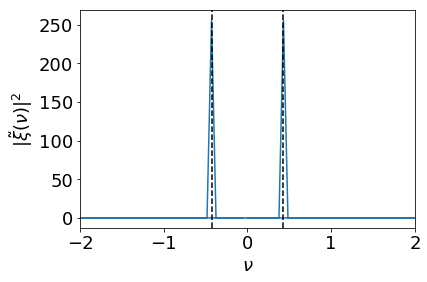### Ornstein–Uhlenbeck process¶

The Ornstein–Uhlenbeck (OU) process is defined by the following Langevin equation $$\dot v = -\gamma v + \eta ~,$$ where $\gamma$ is the friction and $\eta$ is the noise term, obeying $\langle \eta(t) \eta(t+\tau) \rangle = 2\gamma$.

The dynamics is solved with the first order Euler scheme $v_{i+1}=v_i - \gamma v_i \Delta t + \sqrt{2\gamma\Delta t} \chi$ where $\chi$ is a normally sampled number with zero mean and unit variance. $v(t=0)=0$ and 1024 loops of thermalization are performed.

For the OU process, the autocorrelation decays exponentially. The power spectrum is a Lorentzian $$S(\nu) = \frac{\gamma}{\gamma^2 + (2\pi \nu)^2}$$ and must be scaled by the sampling time of the signal.

In :
# First order Euler integration of the Langevin equation

N = 8192
v = 0
dt = 0.03
T = N*dt
time = np.arange(N)*dt
gamma = 2.5
v_factor = math.sqrt(2*gamma*dt)
v_data = []
for t in range(1024):
F = random.gauss(0,1)
v = v - gamma*v*dt + v_factor*F
for t in range(N):
F = random.gauss(0,1)
v = v - gamma*v*dt + v_factor*F
v_data.append(v)
v_data = np.array(v_data)

In :
# Plot the time series for v(t)
plt.plot(time, v_data)
plt.xlabel(r'$t$')
plt.ylabel(r'$v(t)$')
plt.xlim(0, 30/gamma);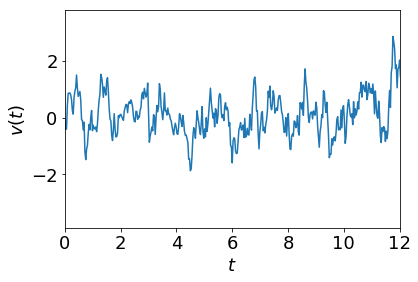In :
# Analytical value of the autocorrelation

plt.plot(time, np.exp(-gamma*time))

# Compute numerically the autocorrelation via a Fourier transform

fft_cor = scipy.signal.fftconvolve(v_data, v_data[::-1])[N-1:]
fft_cor /= (N - np.arange(N))
plt.plot(time, fft_cor, 'k-', lw=2)

# Compute the autocorrelation via the Wiener-Khinchin theorem

psd = np.fft.fft(v_data)*np.conj(np.fft.fft(v_data))/N
C = np.fft.ifft(psd).real

plt.plot(time, C)

plt.xlim(0, 30/gamma)
plt.ylim(-0.05, 1.1)

plt.xlabel(r'$\tau$')
plt.ylabel(r'$C(\tau)$');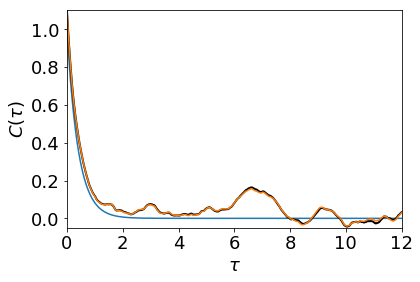In :
# Plot the spectral power density

# Compute the FFT with proper units
t_v = np.fft.fft(v_data)*dt
psd = (t_v*t_v.conjugate()).real
psd[N//2] = np.nan # to avoid the crossing from -infinity to infinity

# The psd is defined per unit time, so 1/T normalizes the result
plt.plot(np.fft.fftfreq(N, dt), psd/T)
# Analytical value
freqs = np.linspace(-2, 2, 100)
plt.plot(freqs, 2*gamma/((2*np.pi*freqs)**2+gamma**2))

plt.xlim(-1, 1)
plt.xlabel(r'$\nu$')
plt.ylabel(r'$S_v(\nu)$');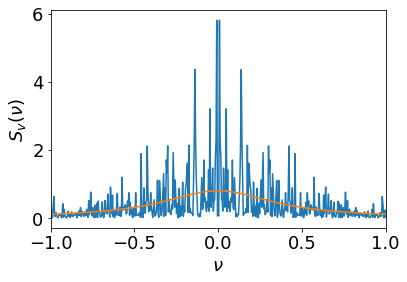## Ending¶

In this post, I presented a short derivation of the Wiener-Kinchin theorem and its numerical application to a periodic signal and to a stochastic process using the scientific Python tools.